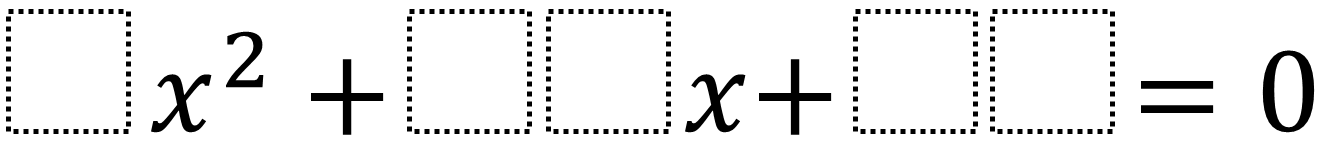Home > High School: Algebra > Factoring Quadratics 2 – Fraction Solution

# Factoring Quadratics 2 – Fraction Solution

Directions: Using the digits 0 to 9 at most one time each, fill in the boxes to so that at least one of the solutions is a fraction.### Hint

How could you find the equation if you already knew the solutions?

### Answer

There are many answers including 9x^2 + 28x + 3 which has solutions of -1/9 and -3.

Source: Daniel Luevanos

## Writing Equivalent Polynomial Expressions

Directions: Use the digits 1-9, at most one time each, to create a true statement. …